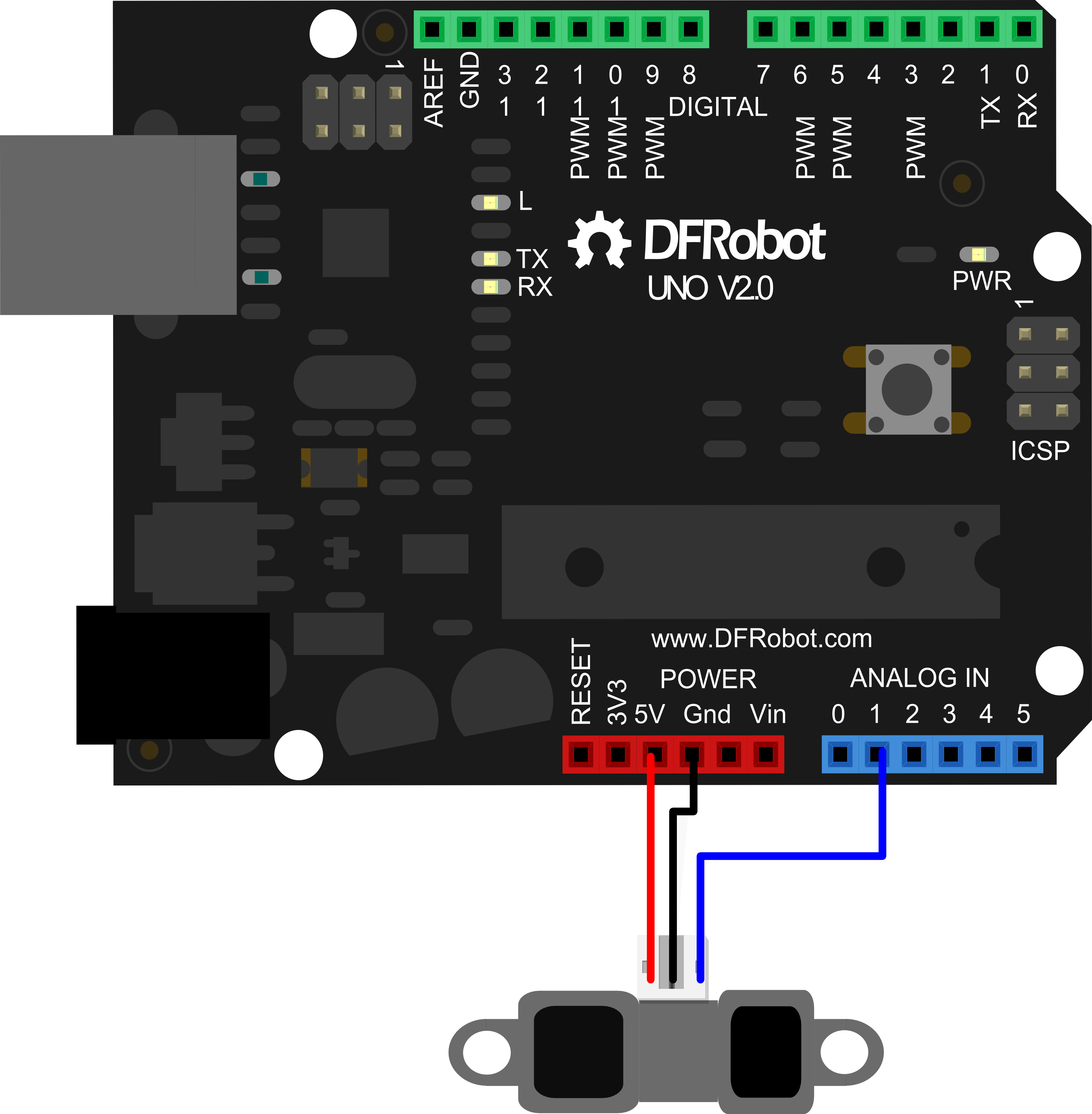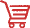Introduction

This SHARP infrared distance sensor takes a continuous distance reading and returns a corresponding analog voltage with a range of 20cm (8") to 150cm (60").It can be usually applied as obstacle avoidance and path planning sensor.

Specifications

• Detection Range :20-150cm
• Voltage :4-5 .5 V
• Current :33-50 mA current consumption
• Output: analog output, the output voltage is proportional to detection range

Connection DiagramSample code

/*
description:
The sample code is used to measure distance by GP2Y0A02YK IR ranger sensor.
VCC -- VCC
GND -- GND
Signal -- Analog 1
*/

int IRpin = 1;                                    // analog pin for reading the IR sensor

void setup() {
Serial.begin(9600);                             // start the serial port
}

void loop() {
float volts = analogRead(IRpin)*0.0048828125;   // value from sensor * (5/1024) - if running 3.3.volts then change 5 to 3.3
float distance = 65*pow(volts, -1.10);          // worked out from graph 65 = theretical distance / (1/Volts)
Serial.println(distance);                       // print the distance
delay(100);                                     // arbitary wait time.
}

DocumentsGet Sharp GP2Y0A02YK IR ranger sensor (150cm) from DFRobot Store or DFRobot Distributor.